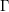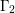Home
Hostname: page-component-568f69f84b-gcfkn Total loading time: 0.235 Render date: 2021-09-19T13:27:03.568Z Has data issue: true Feature Flags: { "shouldUseShareProductTool": true, "shouldUseHypothesis": true, "isUnsiloEnabled": true, "metricsAbstractViews": false, "figures": true, "newCiteModal": false, "newCitedByModal": true, "newEcommerce": true, "newUsageEvents": true }Compositio Mathematica

# The Chowla–Selberg formula for abelian CM fields and Faltings heights

Published online by Cambridge University Press:  24 September 2015

## Abstract

HTML view is not available for this content. However, as you have access to this content, a full PDF is available via the ‘Save PDF’ action button.

In this paper we establish a Chowla–Selberg formula for abelian CM fields. This is an identity which relates values of a Hilbert modular function at CM points to values of Euler’s gamma function${\rm\Gamma}$ and an analogous function${\rm\Gamma}_{2}$ at rational numbers. We combine this identity with work of Colmez to relate the CM values of the Hilbert modular function to Faltings heights of CM abelian varieties. We also give explicit formulas for products of exponentials of Faltings heights, allowing us to study some of their arithmetic properties using the Lang–Rohrlich conjecture.

## MSC classification

Type
Research Article
Information
Compositio Mathematica , March 2016 , pp. 445 - 476

## References

Anderson, G. W., Logarithmic derivatives of Dirichlet L-functions and the periods of abelian varieties, Compositio Math. 45 (1982), 315332.Google Scholar
Anderson, G. W., Brownawell, D. W. and Papanikolas, M. A., Determination of the algebraic relations among special Γ-values in positive characteristic, Ann. of Math. (2) 160 (2004), 237313.CrossRefGoogle Scholar
Asai, T., On a certain function analogous to log|𝜂(z)|, Nagoya Math. J. 40 (1970), 193211.CrossRefGoogle Scholar
Bost, J.-B., Mestre, J.-F. and Moret-Bailly, L., Sur le calcul expliref des ‘classes de Chern’ des surfaces arithmétiques de genre 2 [On the explicit calculation of the ‘Chern classes’ of arithmetic surfaces of genus 2], in Séminaire sur les pinceaux de courbes elliptiques (Paris, 1988), Astérisque, vol. 183 (Société Mathématique de France, 1990), 69105 (in French).Google Scholar
Bruinier, J. H., Kudla, S. S. and Yang, T. H., Special values of Green functions at big CM points, Int. Math. Res. Not. IMRN 9 (2012), 19171967.Google Scholar
Bruinier, J. H. and Yang, T. H., CM-values of Hilbert modular functions, Invent. Math. 163 (2006), 229288.CrossRefGoogle Scholar
Bruinier, J. H. and Yang, T. H., Twisted Borcherds products on Hilbert modular surfaces and their CM values, Amer. J. Math. 129 (2007), 807841.CrossRefGoogle Scholar
Bruinier, J. H. and Yang, T. H., CM values of automorphic Green functions on orthogonal groups over totally real fields, in Arithmetic geometry and automorphic forms, Advanced Lectures in Mathematics (ALM), vol. 19 (International Press, Somerville, MA, 2011), 154.Google Scholar
Chowla, S. and Selberg, A., On Epstein’s zeta function. I, Proc. Natl. Acad. Sci. USA 35 (1949), 371374.CrossRefGoogle ScholarPubMed
Chowla, S. and Selberg, A., On Epstein’s zeta-function, J. reine angew. Math. 227 (1967), 86110.Google Scholar
Cohen, H., Number theory, in Analytic and modern tools, Vol. II, Graduate Texts in Mathematics, vol. 240 (Springer, New York, 2007).Google Scholar
Colmez, P., Périodes des variétés abéliennes à multiplication complexe, Ann. of Math. (2) 138 (1993), 625683.CrossRefGoogle Scholar
Conner, P. E. and Hurrelbrink, J., Class number parity, Series in Pure Mathematics, vol. 8 (World Scientific, Singapore, 1988).CrossRefGoogle Scholar
Deligne, P., Valeurs de fonctions L et périodes d’intégrales, in Automorphic forms, representations and L-functions (Oregon State University, Corvallis, OR, 1977), Part 2, Proceedings of Symposia in Pure Mathematics, vol. XXXIII (American Mathematical Society, Providence, RI, 1979), 313346; with an appendix by N. Koblitz and A. Ogus.Google Scholar
Deninger, C., On the analogue of the formula of Chowla and Selberg for real quadratic fields, J. reine angew. Math. 351 (1984), 171191.Google Scholar
Fröhlich, A., Central extensions, Galois groups, and ideal class groups of number fields, Contemporary Mathematics, vol. 24 (American Mathematical Society, Providence, RI, 1983).CrossRefGoogle Scholar
Gross, B. H., Arithmetic on elliptic curves with complex multiplication, Lecture Notes in Mathematics, vol. 776 (Springer, Berlin, 1980); with an appendix by B. Mazur.CrossRefGoogle Scholar
Gross, B. H. and Zagier, D. B., On singular moduli, J. reine angew. Math. 355 (1985), 191220.Google Scholar
Kato, K., Kurokawa, N. and Saito, T., Number theory. 2. Introduction to class field theory, Translations of Mathematical Monographs, vol. 240, Iwanami Series in Modern Mathematics (American Mathematical Society, Providence, RI, 2011); Translated from the 1998 Japanese original by Masato Kuwata and Katsumi Nomizu.Google Scholar
Lang, S., Cyclotomic fields I and II, Graduate Texts in Mathematics, vol. 121 (Springer, New York, 1990).CrossRefGoogle Scholar
Lang, S., Algebraic number theory, Graduate Texts in Mathematics, vol. 110, second edition (Springer, New York, 1994).CrossRefGoogle Scholar
Lerch, M., Sur quelques formules relatives au nombre des classes, Bull. Sci. Math. (2) 21 (1897), 302303.Google Scholar
Masri, R., CM cycles and nonvanishing of class group L-functions, Math. Res. Lett. 17 (2010), 749760.CrossRefGoogle Scholar
Moreno, C. J., The Chowla–Selberg formula, J. Number Theory 17 (1983), 226245.CrossRefGoogle Scholar
Mouhib, A., On the parity of the class number of multiquadratic number fields, J. Number Theory 129 (2009), 12051211.CrossRefGoogle Scholar
Obus, A., On Colmez’s product formula for periods of CM-abelian varieties, Math. Ann. 356 (2013), 401418.CrossRefGoogle Scholar
Shintani, T., On evaluation of zeta functions of totally real algebraic number fields at non-positive integers, J. Fac. Sci. Univ. Tokyo Sect. IA Math. 23 (1976), 393417.Google Scholar
Shintani, T., On values at s = 1 of certain L functions of totally real algebraic number fields, in Algebraic number theory (Kyoto Int. Sympos., Res. Inst. Math. Sci., Univ. Kyoto, Kyoto, 1976) (Japan Society for the Promotion of Science, Tokyo, 1977), 201212.Google Scholar
Silverman, J. H., Heights and elliptic curves, in Arithmetic geometry (Storrs, CT, 1984) (Springer, New York, 1986), 253265.CrossRefGoogle Scholar
van der Geer, G., Hilbert modular surfaces, in Ergebnisse der Mathematik und ihrer Grenzgebiete (3) [Results in mathematics and related areas (3)], Vol. 16 (Springer, Berlin, 1988).Google Scholar
Weil, A., Elliptic functions according to Eisenstein and Kronecker, in Ergebnisse der Mathematik und ihrer Grenzgebiete, Band 88 (Springer, New York, 1976).Google Scholar
Yang, T. H., An arithmetic intersection formula on Hilbert modular surfaces, Amer. J. Math. 132 (2010), 12751309.CrossRefGoogle Scholar
Yang, T. H., The Chowla–Selberg formula and the Colmez conjecture, Canad. J. Math. 62 (2010), 456472.CrossRefGoogle Scholar
Yang, T. H., Arithmetic intersection on a Hilbert modular surface and the Faltings height, Asian J. Math. 17 (2013), 335381.CrossRefGoogle Scholar
Yoshida, H., On absolute CM-periods, in Automorphic forms, automorphic representations, and arithmetic (Fort Worth, TX, 1996), Proceedings of Symposia in Pure Mathematics, vol. 66, Part 1 (American Mathematical Society, Providence, RI, 1999), 221278.CrossRefGoogle Scholar
Zagier, D., Elliptic modular forms and their applications, in The 1-2-3 of modular forms, Universitext (Springer, Berlin, 2008), 1103.Google ScholarYou have Access
1
Cited by

# Send article to Kindle

Note you can select to send to either the @free.kindle.com or @kindle.com variations. ‘@free.kindle.com’ emails are free but can only be sent to your device when it is connected to wi-fi. ‘@kindle.com’ emails can be delivered even when you are not connected to wi-fi, but note that service fees apply.

Find out more about the Kindle Personal Document Service.

The Chowla–Selberg formula for abelian CM fields and Faltings heights
Available formats
×

# Send article to Dropbox

To send this article to your Dropbox account, please select one or more formats and confirm that you agree to abide by our usage policies. If this is the first time you use this feature, you will be asked to authorise Cambridge Core to connect with your <service> account. Find out more about sending content to Dropbox.

The Chowla–Selberg formula for abelian CM fields and Faltings heights
Available formats
×

# Send article to Google Drive

To send this article to your Google Drive account, please select one or more formats and confirm that you agree to abide by our usage policies. If this is the first time you use this feature, you will be asked to authorise Cambridge Core to connect with your <service> account. Find out more about sending content to Google Drive.

The Chowla–Selberg formula for abelian CM fields and Faltings heights
Available formats
×
×